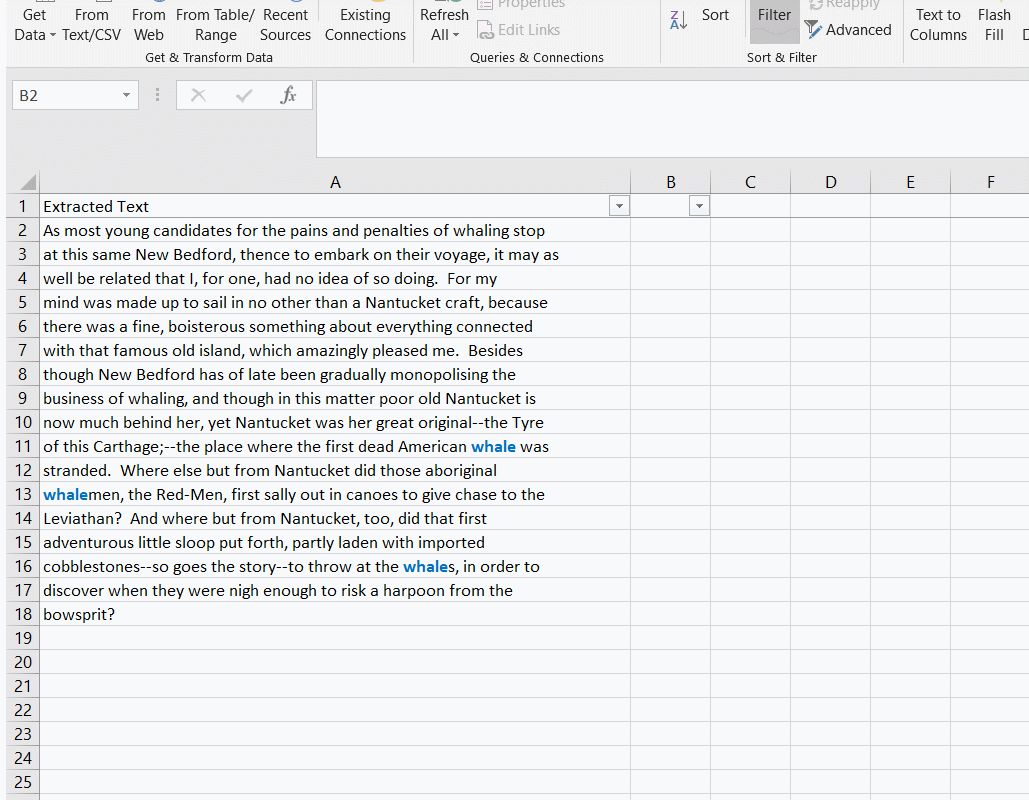top of pageSearch

# Excel formula to check to see if a cell contains a string

The SEARCH formula used in conjunction with the ISNUMBER formula in Excel will allow you to search for a particular string inside the contents of a cell. So in this example the formula:

=IF(ISNUMBER(SEARCH("whale",A2)),A2,B1). . . will search the contents of cell A2 for the string 'whale'. If a result is found, the formula will pull the data from cell A2. If it is not found the result from cell above will be entered. When the formula is pulled down the column will be populated only when a hit is found.

Using this formula has an advantage over simply using the contains option in a filter on the column. If you have raw text for multiple documents, and you want to pull the date for each or the title for each, and there is a unique identifier for those values you can search for (and a break indicating where documents begin and end), you can create an index that will have the searched for value in a column. As shown in this example the date is then directly associated with the Bates number for different documents.bottom of page